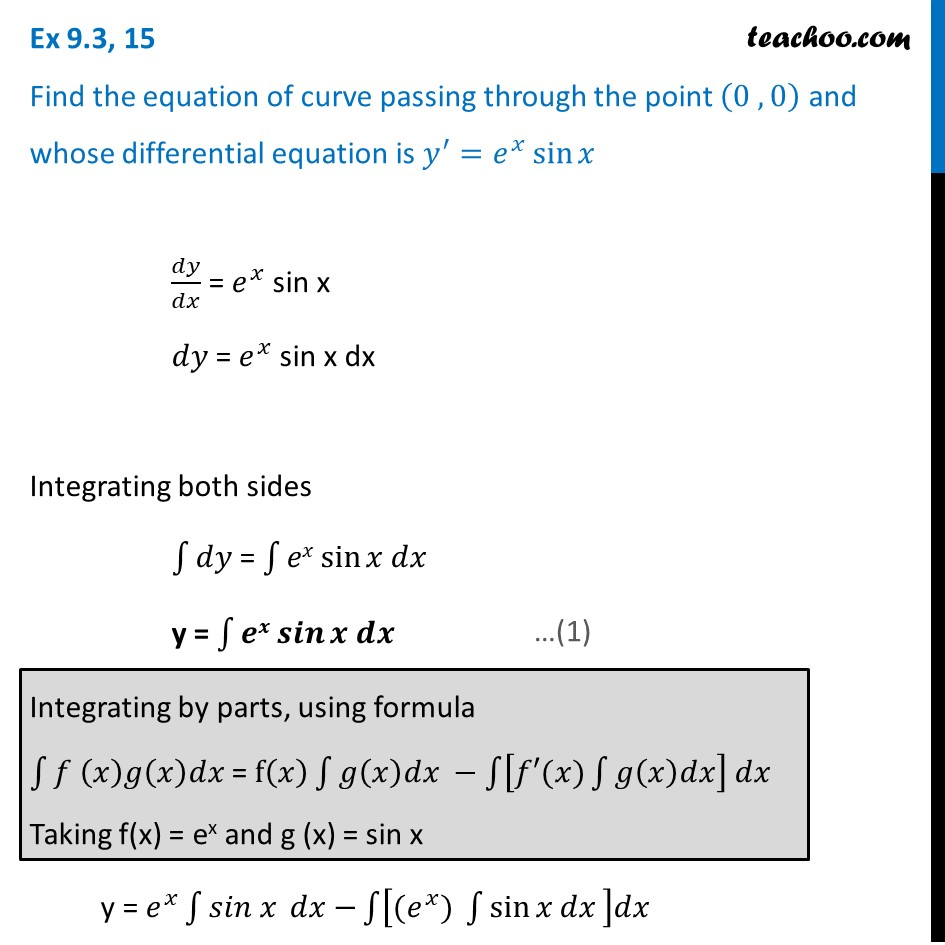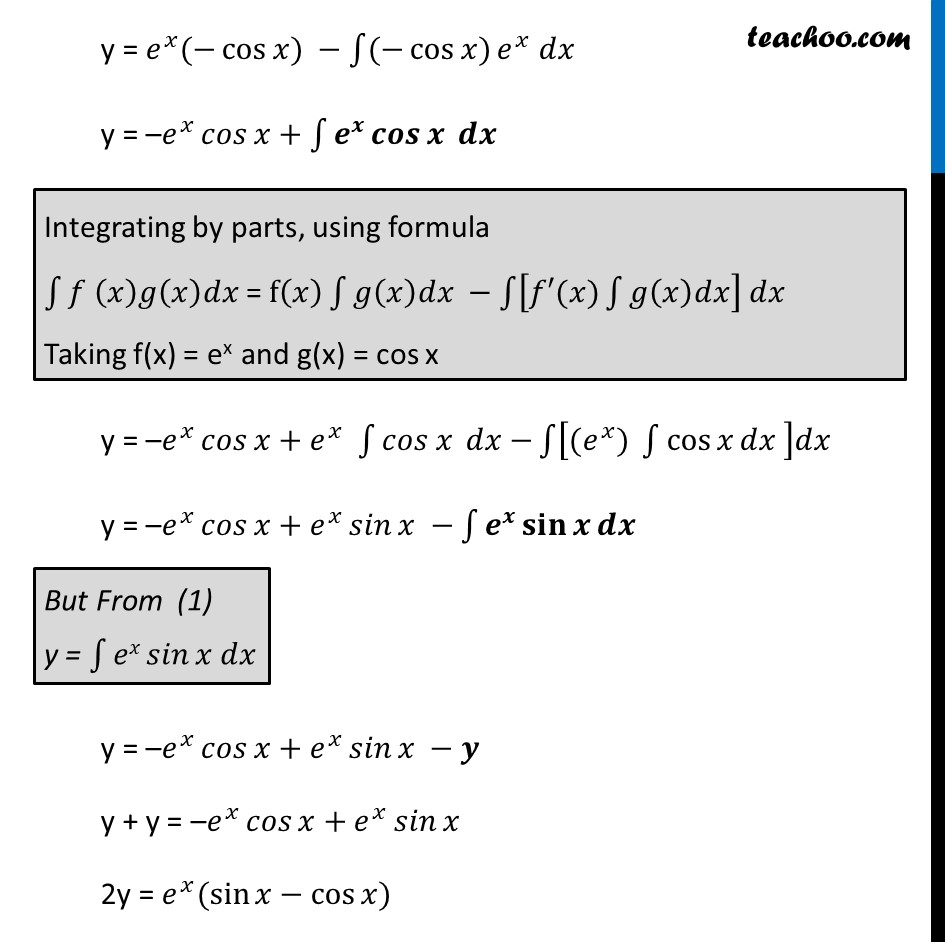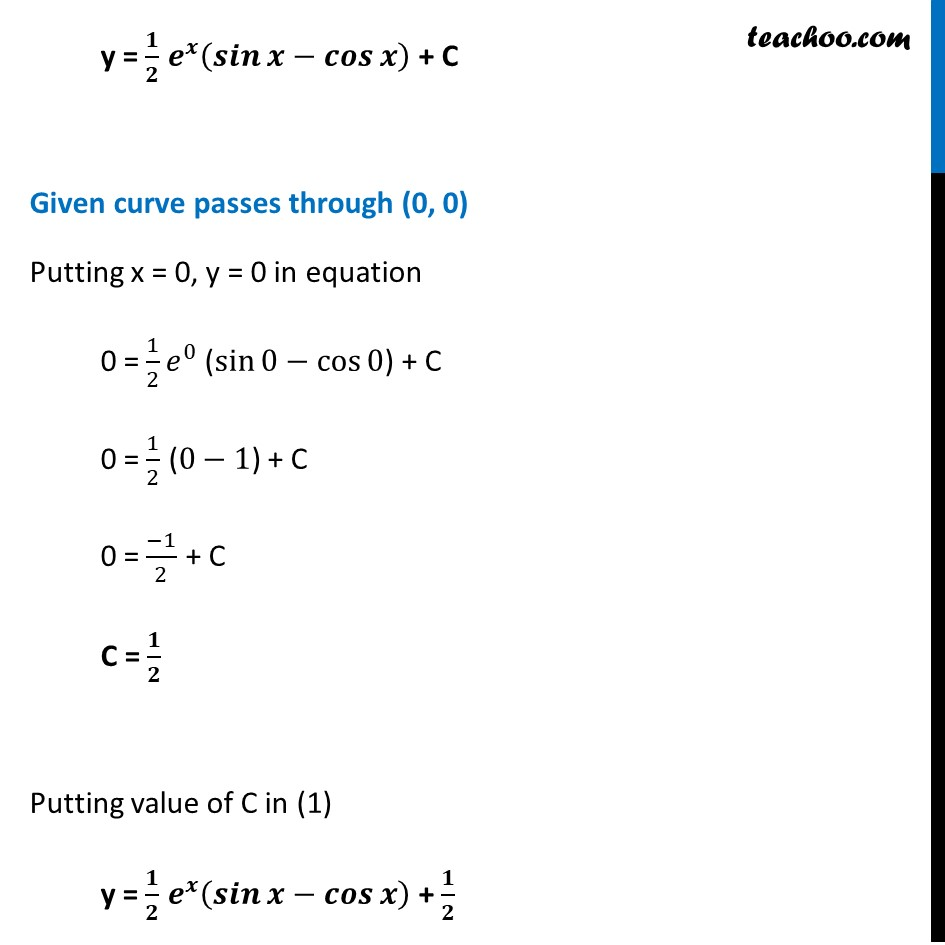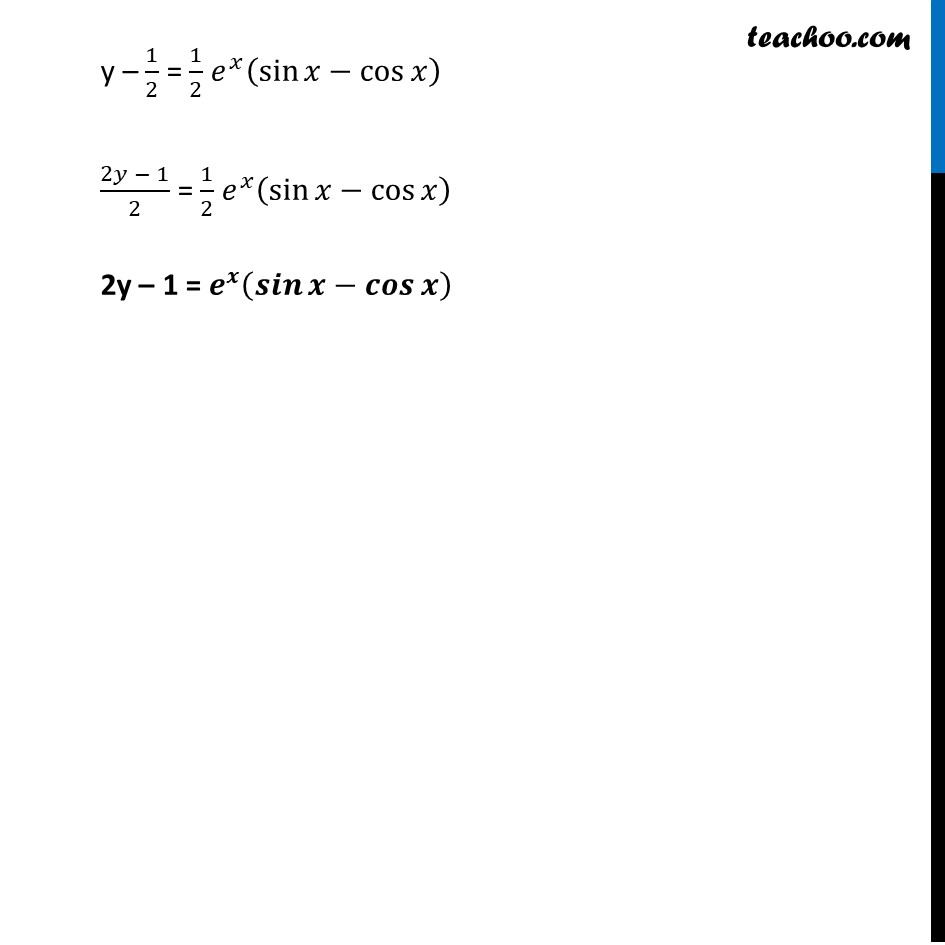Ex 9.3

Chapter 9 Class 12 Differential Equations
Serial order wiseLearn in your speed, with individual attention - Teachoo Maths 1-on-1 Class

### Transcript

Ex 9.3, 15 Find the equation of curve passing through the point (0 , 0) and whose differential equation is 𝑦^′=𝑒^𝑥 sin⁡𝑥 𝑑𝑦/𝑑𝑥 = 𝑒^𝑥 sin x 𝑑𝑦 = 𝑒^𝑥 sin x dx Integrating both sides ∫1▒𝑑𝑦 = ∫1▒〖𝑒𝑥 sin⁡〖𝑥 𝑑𝑥〗 〗 y = ∫1▒〖𝒆𝒙 𝒔𝒊𝒏⁡〖𝒙 𝒅𝒙〗 〗 Integrating by parts, using formula ∫1▒〖𝑓 (𝑥)𝑔(𝑥)𝑑𝑥 〗= f(𝑥) ∫1▒〖𝑔(𝑥)𝑑𝑥 −∫1▒〖[𝑓′(𝑥)∫1▒𝑔(𝑥)𝑑𝑥] 𝑑𝑥 〗 〗 Taking f(x) = ex and g (x) = sin x y = 𝑒^𝑥 (−cos⁡𝑥) − ∫1▒〖(−cos⁡𝑥)〗 𝑒^𝑥 𝑑𝑥 y = –𝑒^𝑥 𝑐𝑜𝑠⁡𝑥+∫1▒〖𝒆^𝒙 𝒄𝒐𝒔⁡𝒙 𝒅𝒙〗 Integrating by parts, using formula ∫1▒〖𝑓 (𝑥)𝑔(𝑥)𝑑𝑥 〗= f(𝑥) ∫1▒〖𝑔(𝑥)𝑑𝑥 −∫1▒〖[𝑓′(𝑥)∫1▒𝑔(𝑥)𝑑𝑥] 𝑑𝑥 〗 〗 Taking f(x) = ex and g(x) = cos x y = –𝑒^𝑥 𝑐𝑜𝑠⁡𝑥+ 𝑒^𝑥 ∫1▒〖〖𝑐𝑜𝑠 𝑥〗⁡〖 𝑑𝑥〗−〗 ∫1▒[(𝑒^𝑥) ∫1▒〖cos⁡𝑥 𝑑𝑥 〗]𝑑𝑥 y = –𝑒^𝑥 𝑐𝑜𝑠⁡𝑥+𝑒^𝑥 𝑠𝑖𝑛⁡𝑥 −∫1▒〖𝒆^𝒙 𝐬𝐢𝐧⁡𝒙 𝒅𝒙〗 But From (1) y = ∫1▒〖𝑒𝑥 𝑠𝑖𝑛⁡〖𝑥 𝑑𝑥〗 〗 y = –𝑒^𝑥 𝑐𝑜𝑠⁡𝑥+𝑒^𝑥 𝑠𝑖𝑛⁡𝑥 −𝒚 y + y = –𝑒^𝑥 𝑐𝑜𝑠⁡𝑥+𝑒^𝑥 𝑠𝑖𝑛⁡𝑥 2y = 𝑒^𝑥 (sin⁡𝑥−cos⁡𝑥) y = 𝑒^𝑥 ∫1▒〖〖𝑠𝑖𝑛 𝑥〗⁡〖 𝑑𝑥〗−〗 ∫1▒[(𝑒^𝑥) ∫1▒〖sin⁡𝑥 𝑑𝑥 〗]𝑑𝑥 y = 𝟏/𝟐 𝒆^𝒙 (𝒔𝒊𝒏⁡𝒙−𝒄𝒐𝒔⁡𝒙 ) + C Given curve passes through (0, 0) Putting x = 0, y = 0 in equation 0 = 1/2 𝑒^0 (sin⁡0−cos⁡0) + C 0 = 1/2 (0−1) + C 0 = (−1)/2 + C C = 𝟏/𝟐 Putting value of C in (1) y = 𝟏/𝟐 𝒆^𝒙 (𝒔𝒊𝒏⁡𝒙−𝒄𝒐𝒔⁡𝒙 ) + 𝟏/𝟐 y – 1/2 = 1/2 𝑒^𝑥 (sin⁡𝑥−cos⁡𝑥 ) (2𝑦 − 1)/2 = 1/2 𝑒^𝑥 (sin⁡𝑥−cos⁡𝑥 ) 2y – 1 = 𝒆^𝒙 (𝒔𝒊𝒏⁡𝒙−𝒄𝒐𝒔⁡𝒙 )